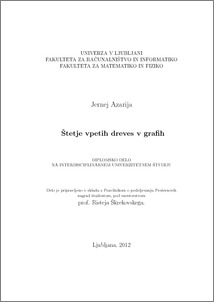# Counting spanning trees in graphs

Jernej Azarija (2012) Counting spanning trees in graphs. Prešeren awards for students.Preview
PDF

## Abstract

The history of counting the number of spanning trees dates back into the year 1842 in which the German mathematician Gustav Kirchhoff derived a relation between the number of spanning trees of a graph G (τ(G)) and the determinant of a specific submatrix associated with G. After this result many other related results followed. For example in 1889 the British mathematician A. Cayley showed that the complete graph on n nodes has nn−2 spanning trees.The function τ can nowadays be found in the field of physics where the number of spanning trees is used as an invariant for computing the entropy of certain networks related to physical processes. One can also find τ(G) in the field of network analysis where τ(G) is used in relation with various quantities indicating the reliability of a network represented by a graph G. In addition there are various applications of the number of spanning trees within mathematics as well. For example, the currently fastest algorithm for embedding a 3-connected planar graph as a 3D polytope uses τ(G) for computing the scaling factor of the coordinate system.In this work we present the function τ and some results related to determining the number of spanning trees of a given graph. In later chapters we will be mainly interested in the question of how large can a class of graphs of order n be if they have mutually distinct number of spanning trees. In addition we will introduce an extremal problem asking for the smallest graph G having a prescribed number of spanning trees.

Item Type: Thesis (Prešeren awards for students)
Keywords: number of spanning trees, graph theory, combinatorial counting
Number of Pages: 44
Language of Content: Slovenian
Mentor / Comentors:
Name and SurnameIDFunction
izr. prof. dr. Riste Škrekovski1489Mentor
Link to COBISS: http://www.cobiss.si/scripts/cobiss?command=search&base=51012&select=(ID=9542228)
Institution: University of Ljubljana
Department: Faculty of Computer and Information Science
Item ID: 3699
Date Deposited: 21 Dec 2016 11:58